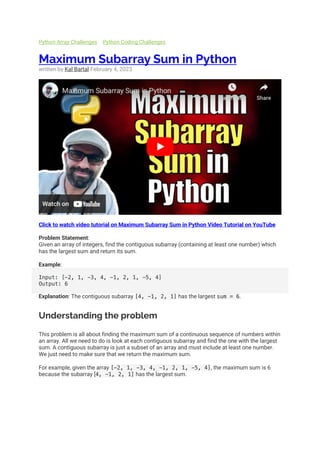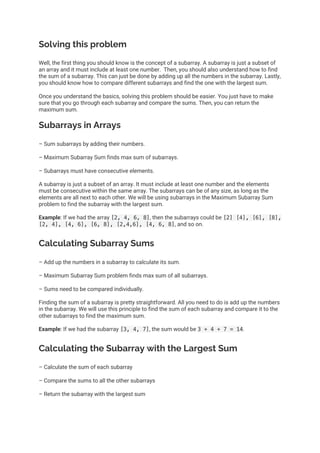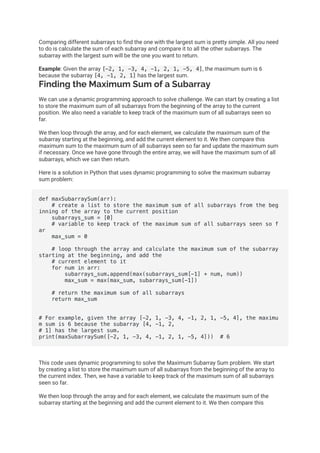Successfully reported this slideshow.

# Maximum Subarray Sum in Python×

# Maximum Subarray Sum in Python

Solve Max Subarray Sum in Python w/ Dynamic Programming for O(n) time/space. Understand problem/subarrays, calculate sums & compare to find max sum. Use list to store subarrays, variable to track max sum seen. Think through code & talk prob. to ensure understanding.

Solve Max Subarray Sum in Python w/ Dynamic Programming for O(n) time/space. Understand problem/subarrays, calculate sums & compare to find max sum. Use list to store subarrays, variable to track max sum seen. Think through code & talk prob. to ensure understanding.